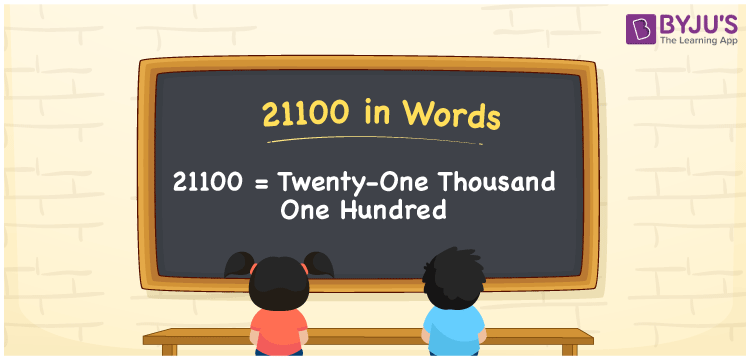# 21100 in words

21100 in words is written as Twenty One Thousand One Hundred. In 21100, 2 has a place value of ten thousand, 1 is in the place value of thousand and the second 1 is in the place value of hundred. The article on Place Value gives more information. The number 21100 is used in expressions that relate to money, distance, social media views, and many more. For example, “I have to save Twenty One Thousand One Hundred Rupees more to buy a gold necklace”.

 21100 in words Twenty One Thousand One Hundred Twenty One Thousand One Hundred in Numbers 21100

## 21100 in English Words## How to Write 21100 in Words?

We can convert 21100 to words using a place value chart. The number 21100 has 5 digits, so let’s make a chart that shows the place value up to 5 digits.

 Ten thousand Thousands Hundreds Tens Ones 2 1 1 0 0

Thus, we can write the expanded form as:

2 × Ten thousand + 1 × Thousand + 1 × Hundred + 0 × Ten + 0 × One

= 2 × 10000 + 1 × 1000 + 1 × 100 + 0 × 10 + 0 × 1

= 21100.

= Twenty One Thousand One Hundred.

21100 is the natural number that is succeeded by 21099 and preceded by 21101.

21100 in words – Twenty One Thousand One Hundred.

Is 21100 an odd number? – No.

Is 21100 an even number? – Yes.

Is 21100 a perfect square number? – No.

Is 21100 a perfect cube number? – No.

Is 21100 a prime number? – No.

Is 21100 a composite number? – Yes.

## Solved Example

1. Write the number 21100 in expanded form

Solution: 2 x 10000 + 1 x 1000 + 1 x 100 + 0 x 10 + 0 x 1

Or Just 2 x 10000 + 1 x 1000 + 1 x 100

We can write 21100 = 20000 + 1000 + 100 + 0 + 0

= 2 x 10000 + 1 x 1000 + 1 x 100 + 0 x 10 + 0 x 1.

## Frequently Asked Questions on 21100 in words

Q1

### How to write the number 21100 in words?

21100 in words is written as Twenty One Thousand One Hundred.
Q2

### Is 21100 divisible by 3?

No. 21100 is not divisible by 3.
Q3

### Is 21100 divisible by 10?

Yes. 21100 is divisible by 10.